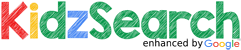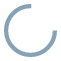### more

facts wiki news games kidztube apps

In mathematics, the word "division" means the operation which is the opposite of multiplication. Some symbols for division can be a slash (/), a (fraction) line, or the division sign (/), as in:

6/3\,    or    \frac 63    or    6 / 3.

where each of the three expressions means "6 divided by 3", with 2 as the answer. The first number is the dividend (6), and the second number is the divisor (3). The result (or answer) is the quotient, where any left-over amount as whole numbers is called the "remainder". For example, 14/4 gives quotient 3 with remainder 2, all expressed as the number 324, or as 312 or 3.5). view more...report a search problem